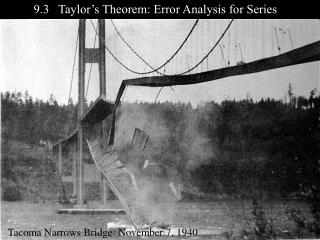DownloadDownload Presentation9.3 Taylor’s Theorem: Error Analysis for Series

# 9.3 Taylor’s Theorem: Error Analysis for Series

Télécharger la présentation## 9.3 Taylor’s Theorem: Error Analysis for Series

- - - - - - - - - - - - - - - - - - - - - - - - - - - E N D - - - - - - - - - - - - - - - - - - - - - - - - - - -
##### Presentation Transcript

1. 9.3 Taylor’s Theorem: Error Analysis for Series Tacoma Narrows Bridge: November 7, 1940

2. Last time in BC…

3. So the Taylor Series for ln x centered at x = 1 is given by… Use the first two terms of the Taylor Series for ln x centered at x = 1 to approximate:

4. Recall that the Taylor Series for ln x centered at x = 1 is given by… Find the maximum error bound for each approximation. Because the series is alternating, we can start with… Wait! How is the actual error bigger than the error bound for ln 0.5?

5. And now, the exciting conclusion of Chapter 9…

6. Since each term of a convergent alternating series moves the partial sum a little closer to the limit: Alternating Series Estimation Theorem For a convergent alternating series, the truncation error is less than the first missing term, and is the same sign as that term. This is also a good tool to remember because it is easier than the Lagrange Error Bound…which you’ll find out about soon enough… Muhahahahahahaaa!

7. Taylor’s Theorem with Remainder If f has derivatives of all orders in an open interval I containing a, then for each positive integer n and for each x in I: Lagrange Error Bound In this case, c is the number between x and athat will give us the largest result for

8. Does any part of this look familiar? If our Taylor Series had alternating terms: If our Taylor Series did not have alternating terms: This remainder term is just like the Alternating Series error (note that it uses the n + 1 term) except for the Note that working with This is just the next term of the series which is all we need if it is an Alternating Series is the part that makes the Lagrange Error Bound more complicated.

9. Taylor’s Theorem with Remainder If f has derivatives of all orders in an open interval I containing a, then for each positive integer n and for each x in I: Lagrange Error Bound Why this is the case involves a mind-bending proof so we just won’t do it here. Now let’s go back to our last problem…

10. Recall that the Taylor Series for ln x centered at x = 1 is given by… Find the maximum error bound for each approximation. Because the series is alternating, we can start with… Wait! How is the actual error bigger than the error bound for ln 0.5?

11. Recall that the Taylor Series for ln x centered at x = 1 is given by… First of all, when plugging in ½ for x, what happens to your series? Note that when x = ½, the series is no longer alternating. So now what do we do? Since the Remainder Term will work for any Taylor Series, we’ll have to use it to find our error bound in this case

12. Since we used terms up through n = 2, we will need to go to n = 3 to find our Remainder Term(error bound): The Taylor Series for centered at x = 1 The third derivative gives us this coefficient: This is the part of the error bound formula that we need

13. We saw that plugging in ½ for x makes each term of the series positive and therefore it is no longer an alternating series. So we need to use the Remainder Term which is also called… The Lagrange Error Bound The third derivative of ln x at x = c What value of c will give us the maximum error? Normally, we wouldn’t care about the actual value of c but in this case, we need to find out what value of c will give us the maximum value for 2c–3.

14. The third derivative of ln x at x = c The question is what value of c between x and a will give us the maximum error? So we are looking for a number forcbetween 0.5 and 1. Let’s rewrite it as which has its largest value when c is smallest. c = 0.5 And therefore… Which is larger than the actual error! And we always want the error bound to be larger than the actual error

15. Let’s try using Lagrange on an alternating series We know that since this is an alternating series, the error bound would be But let’s apply Lagrange (which works on all Taylor Series)… The third derivative of ln(1+ x) is The value of cthat will maximize the error is 0 so… Which is the same as the Alternating Series error bound

16. If M is the maximum value of on the interval between a and x, then: Most text books will describe the error bound two ways: Lagrange Form of the Remainder: and Remainder Estimation Theorem: Note from the way that it is described above that M is just another way of saying that you have to maximize Remember that the only difference you need to worry about between Alternating Series error and La Grange is finding